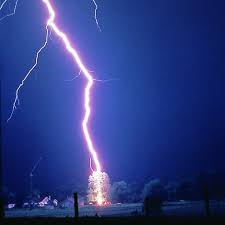# Lightning 69734

The sound travels at a speed of 1km in 3 seconds. If the thunder is heard 12 seconds after the lightning, what distance is the storm?

x =  4 km

### Step-by-step explanation:Did you find an error or inaccuracy? Feel free to write us. Thank you!

Tips for related online calculators
Need help calculating sum, simplifying, or multiplying fractions? Try our fraction calculator.
Check out our ratio calculator.
Do you want to convert velocity (speed) units?
Do you want to convert time units like minutes to seconds?